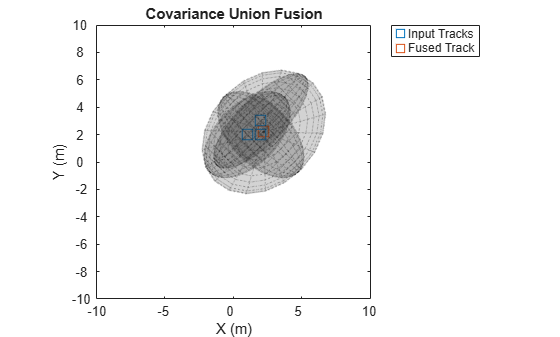# fusecovunion

Covariance fusion using covariance union

## Syntax

``[fusedState,fusedCov] = fusecovunion(trackState,trackCov)``

## Description

example

````[fusedState,fusedCov] = fusecovunion(trackState,trackCov)` fuses the track states in `trackState` and their corresponding covariance matrices `trackCov`. The function estimates the fused state and covariance in a way that maintains consistency. For more details, see Consistent Estimator.```

## Examples

collapse all

Define a state vector of tracks.

```x(:,1) = [1;2;0]; x(:,2) = [2;2;0]; x(:,3) = [2;3;0];```

Define the covariance matrices of the tracks.

```p(:,:,1) = [10 5 0; 5 10 0;0 0 1]; p(:,:,2) = [10 -5 0; -5 10 0;0 0 1]; p(:,:,3) = [12 9 0; 9 12 0;0 0 1];```

Estimate the fused state vector and its covariance.

`[fusedState,fusedCov] = fusecovunion(x,p);`

Use `trackPlotter` to plot the results.

```tPlotter = theaterPlot('XLim',[-10 10],'YLim',[-10 10],'ZLim',[-10 10]); tPlotter1 = trackPlotter(tPlotter, ... 'DisplayName','Input Tracks','MarkerEdgeColor',[0.000 0.447 0.741]); tPlotter2 = trackPlotter(tPlotter, ... 'DisplayName','Fused Track','MarkerEdgeColor',[0.850 0.325 0.098]); plotTrack(tPlotter1,x',p) plotTrack(tPlotter2, fusedState', fusedCov) title('Covariance Union Fusion')```## Input Arguments

collapse all

Track states, specified as an N-by-M matrix, where N is the dimension of the state and M is the number of tracks.

Data Types: `single` | `double`

Track covariance matrices, specified as an N-by-N-by-M array, where N is the dimension of the state and M is the number of tracks.

Data Types: `single` | `double`

## Output Arguments

collapse all

Fused state, returned as an N-by-1 vector, where N is the dimension of the state.

Fused covariance matrix, returned as an N-by-N matrix, where N is the dimension of the state.

collapse all

### Consistent Estimator

A consistent estimator is an estimator that converges in probability to the quantity being estimated as the sample size grows. In the case of tracking, a position estimate is consistent if its covariance (error) matrix is not smaller than the covariance of the actual distribution of the true state about the estimate. The covariance union method guarantees consistency by ensuring that all the individual means and covariances are bounded by the fused mean and covariance.

 Reece, Steven, and Stephen Rogers. "Generalised Covariance Union: A Unified Approach to Hypothesis Merging in Tracking." IEEE® Transactions on Aerospace and Electronic Systems. Vol. 46, No. 1, Jan. 2010, pp. 207–221.

##### SupportGet trial now